# AP Calculus AB Free Response Practice Problems

One of the best ways to study for the AP Calculus Free Response Questions is to practice with as many AP exam style problems as you can.

Here is a handful of AP Calculus Free Response style practice problems that you can work through, along with the full solutions. These only represent a small fraction of the types of questions that might come up on an AP Calculus AB Exam; the more problems you can do before the exam, the better prepared you will be.

## AP Calculus AB Exam Free Response Practice Problems

#### 1. An object moves back and forth across the x-axis. Its velocity can be modeled by the equation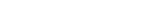. At time t=10, the car is at a position x = 5. [Calculator required] a) What is the total displacement of the car between the times t = 0 and t=5 b) At time t = 10, is the car slowing down, or speeding up? c) What is the position of the particle at the initial time? d) Find a time when the velocity equal to 0.

Solution:
a) Whenever we have a velocity question, we should think back to the following relationships:The total displacement therefore in the integral of the velocity function between the bounds givenThis is an integral that can easily be done by hand. However you should learn how to use you graphing calculator to do this question because many integrals will be far more difficult.

b) Slowing down and speeding up are questions about whether or not the acceleration is positive or negative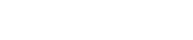cos(t) is negative when t = 10. Therefore, the object is slowing down.

c) Now we need to take the indefinite integral to get a function of position. Do not forget to solve for our constant.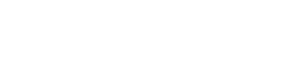To solve for c, we need to know one point: At time t=10, the car is at a position x = 5.The initial time, t=0 gives us a position: x(0) = 0-cos(0)-.839 = -1.839

d) Simply find a root for our initial equation for velocity. t = 5.76 is one of several possibilities.

#### 2. Below is the graph of the g(x). [No Calculator]#### If g(x) = f'(x) a) Where does f(x) have a relative minimum? b) Where does f(x) have a relative maximum? c) If f(x) has an inflection point, approximately where does it lie?

Solution:

a) This function has a relative minimum at x = 0. We should see that it is a minimum because the derivative goes from negative to positive at this point.
b) This function has a relative maximum at x = -2.5 We should see that it is a maximum because the derivative goes from positive to negative at this point.
c) The inflection points exist where the second derivative is 0. We should see this in the graph as existing around x= – 1.25, x = 2, and x = 4

#### 3. We need to enclose an area within a fence. We have 50 feet of fencing available, and a permanent straight wall of infinite length exists on one side (therefore not needing fencing). What dimensions of the fence give the largest possible area?

Solution:

This is a quintessential optimization problem. It is often a good idea to sketch the situation given. Visualizing the problem often helps in finding the correct governing equations. AP exams sometimes do this for you.The variables in this problem are the lengths of x and y. These are the only two properties which change.

Optimization problem will almost always have two functions involved; in this case the two functions involve area and perimeter.We know that Perimeter P = 50

We need to maximize A = xy with the constraint 50 = x + 2y.

We can rewrite the constraint as one function of another. We put this into the area equation to give:At this point, we should go back to the constraint and figure out the domain of y. In this case, with 50m of fence, y=25m. We will come back to this later.

We are asked to find the maximum value of A. As in all maximum/minimum value problems, we find the derivative.We set this equal to 0 and solve for y. In this case, y = 12.5. If we put these numbers back into the constraint:

50 = x + 2y

x = 25

We are asked for dimensions — The fence is 25m x 12.5m

#### 4. Let’s say we want to make a three-dimensional box with a square base (l=w), but we want the surface area to be no greater than 10 m^3. What are the dimensions for a box with the maximum volume?

Solution:

This problem is an optimization problem, similar to the last one. If we’re ever not sure, first step is always to draw out the situation:Two governing equations:

Equation 1: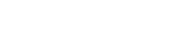Equation 2: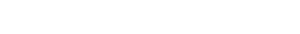We know w = l, so we can use this to simply the first two equations: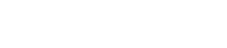We now have a two variable problem:Plug into Volume EquationAny maximization or minimization, we are going to take the derivative and set to zero. In this case, take derivative of V and set to 0.We can throw out the negative case because it has no physical meaning in this problem.  Always remember, if a question is asking something about the physical world, ask yourself the question ‘does the answer make sense?’  If you get answers that seem ridiculous, go back over your work.The box is a cube.

## Final Thoughts

These four questions represent just a small fraction of the possible types of questions that can be asked on the AP exam. Check back later to see additional sample multiple choice questions, related rates questions, as well as comprehensive reviews of a ton of other topics that you might see on the AP Calculus exam.

#### Improve your SAT or ACT score, guaranteed. Start your 1 Week Free Trial of Magoosh SAT Prep or your 1 Week Free Trial of Magoosh ACT Prep today!## Author

•Zachary is a former mechanical engineer and current high school physics, math, and computer science teacher. He graduated from McGill University in 2011 and spent time in the automotive industry in Detroit before moving into education. He has been teaching and tutoring for the past five years, but you can also find him adventuring, reading, rock climbing, and traveling whenever the opportunity arises.